# Current used in calculations as Current Density

When defining the electromagnetic condition "current" I think that AIM is actually using the value as current density!

When I enter 2 Mega Amps (yes 2,000,000 Amps) my simulation yields a current density of 15915816 Amps/m^2 in my copper vias. Yielding a total current of 2 Amps (should be 2,000,000 Amps). See my calculations and images below

# Of Copper vias: 4

Diameter of 1 Copper via = 200 um = 0.0002 m

Radius of 1 copper via = 0.0001 m

Area of 1 Copper via = 3.14159 * 0.0001*0.0001 = 0.0000000314159 m^2

Current = current density * Total Area = 0.0000000314159 m^2 * 4 * 15915816 A/m^2 = 2 A

***NOT EQUIVALENT TO THE 2,000,000 AMPS I ENTERED****

Therefore you have to enter current density for a known surface area to define the current you want!

Either the units for the current need to be changed to Amps per unit area, or the calculation needs to change to allow the user to input desired current values!

Can anyone else validate this?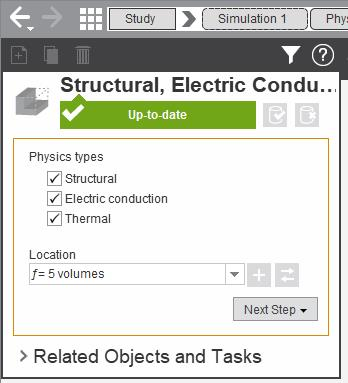Defining current under electromagnetic conditions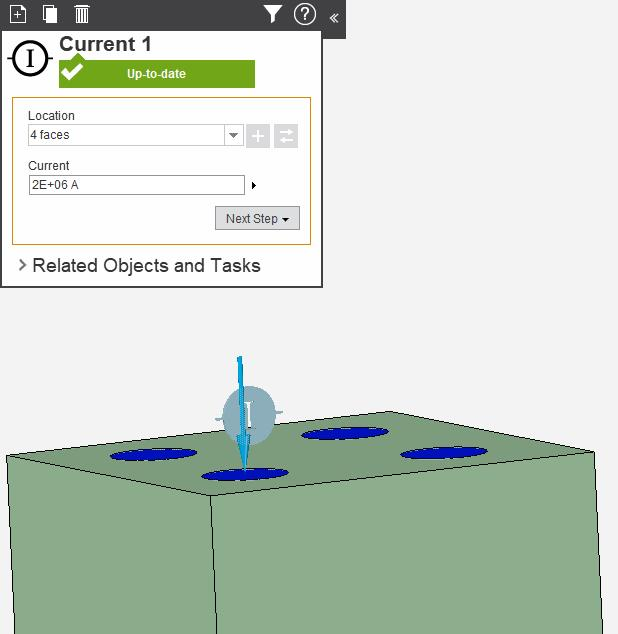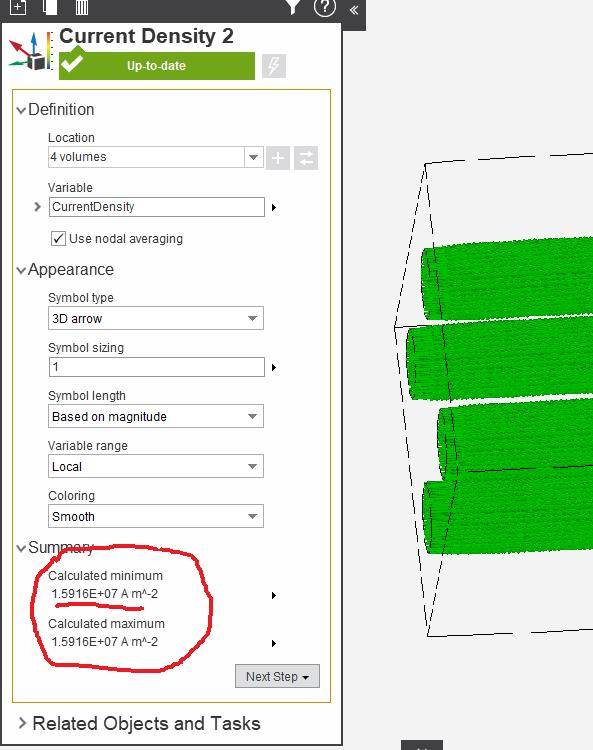• Portland, Oregon, USAMember
edited July 2017

I could be way off here, but in the final screenshot you can see the four cylindrical via volumes selected. The calculated current density is showing the min & max for the currently selected volumes. What happens if you change the location to the 4 areas of interest instead?

• By "area of interest" I assume you mean the faces?

I get the same min and max values whether I select one face or 4: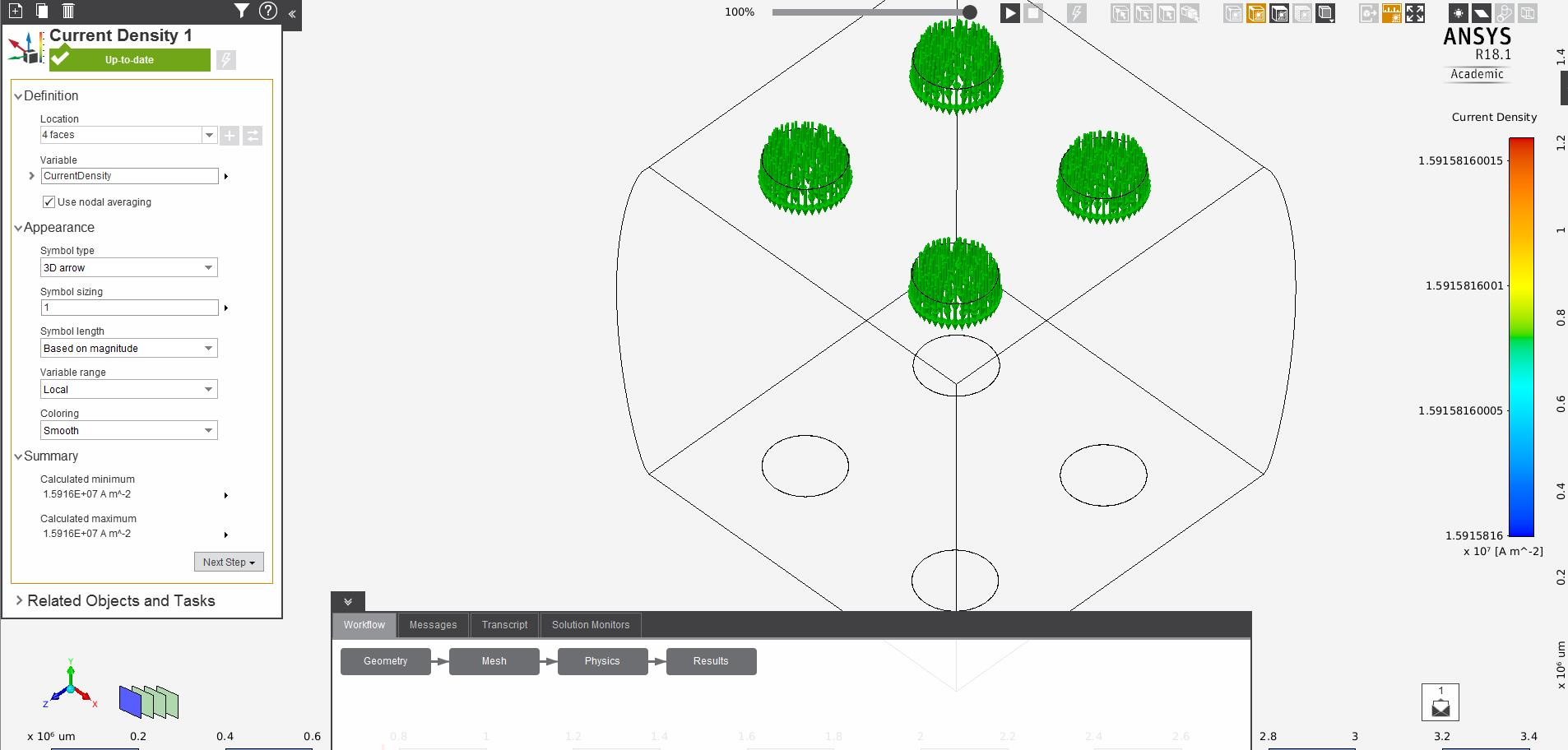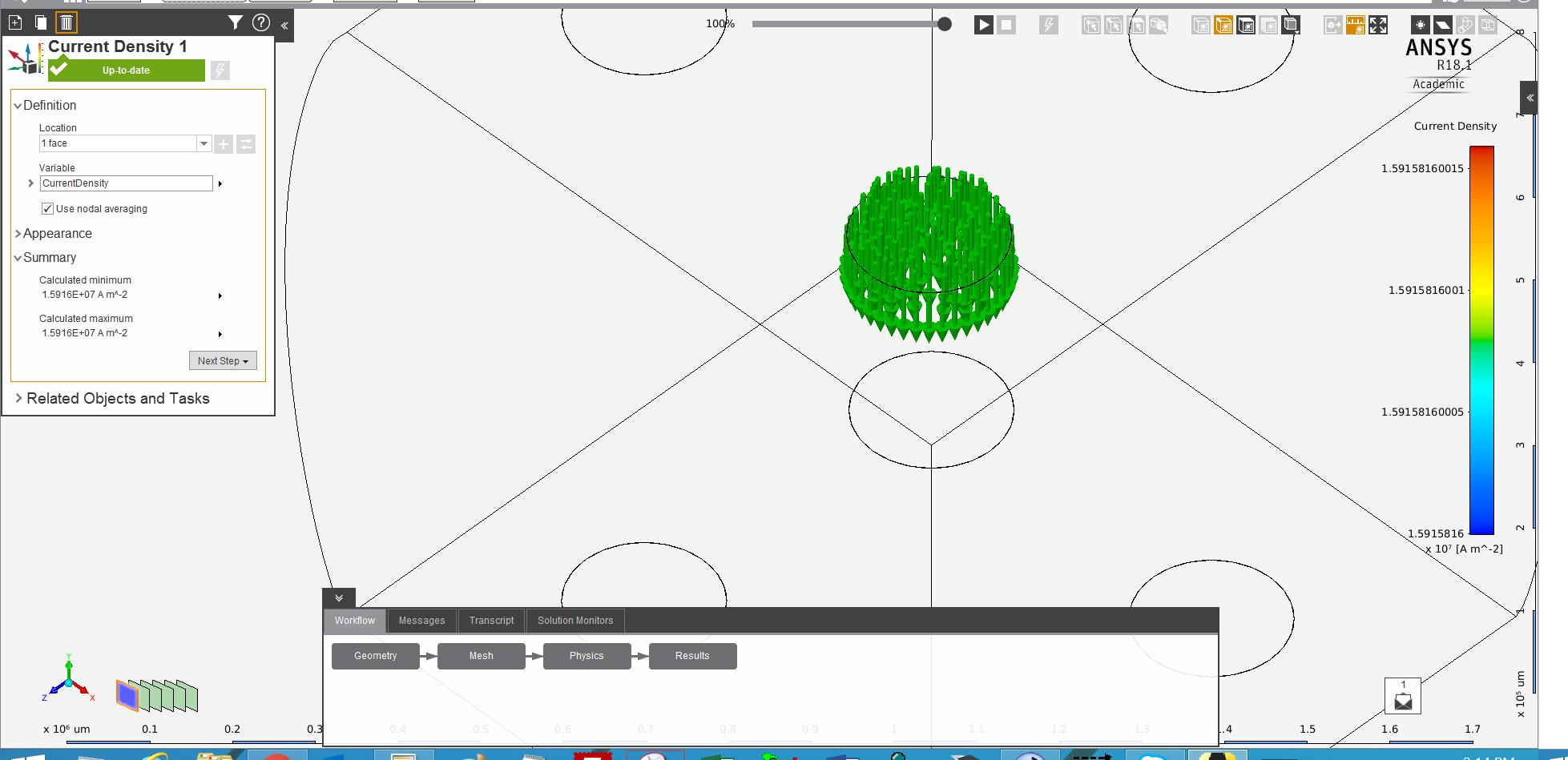• Portland, Oregon, USAMember
edited July 2017

Yes that's exactly what I meant. O.K. Let's see if the rest of the Student Community can chime in here to help out.